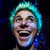## Moonshine E​.​P

### by Earthworm Jim + Spongebob Squarewave

supported byNick Drexler i love 200 bpm, but its nice to see spongebob stuff at 170! SOMEBODY IS SOOOOO GOOOD! Big ups on Run Away too. <3<3<3<3<3<3<3<3<3<3<3<3<3<3<3<3<3<3<3<3<3<3<3<3<3<3<3<3<3<3<3<3<3<3<3<3<3<3<3<3<3<3<3<3<3<3<3<3<3<3<3<3<3<3<3<3<3<3<3<3<3<3<3<3<3<3<3<3<3<3<3<3<3<3<3<3<3<3<3<3<3<3<3<3<3<3<3<3<3<3<3<3<3<3<3<3<3<3<3<3<3<3<3<3<3<3<3<3<3<3<3<3<3<3<3<3<3<3<3<3<3<3<3<3<3<3<3<3<3<3<3<3<3<3<3<3<3<3<3<3<3<3<3<3<3<3<3<3<3<3<3<3<3<3<3<3<3<3<3<3<3<3<3<3<3<3<3<3<3<3<3<3<3<3<3<3<3<3<3<3<3<3<3<3<3<3<3<3<3<3<3<3<3<3! Favorite track: Somebody.
more...
 /

Includes unlimited streaming via the free Bandcamp app, plus high-quality download in MP3, FLAC and more.
• ### Full Digital Discography

Get all 168 Off Me Nut releases available on Bandcamp and save 75%.

Includes unlimited streaming via the free Bandcamp app, plus high-quality downloads of The House Of The Sloth, J1T SH*T, Shiver Me Timbres, J69 - Alien Dawg, Seven Kings, Can't Control My Feet, Queue Tantrum, Wave Ur Hands Like, , and , .

 1 The Condition 05:36 2 Run Away 06:18 3 So Hard 05:22 4 Nothin' Can Stop Us 05:55 5 Somebody 06:00

Earthworm Jim and Spongebob Squarewave have teamed up to make this 5 track super-stomper EP ! Straight up bangers on this one , old skool happy hardcore with a new flavour . Brewin' up that bootleg moonshine for the dancefloor ;)

OMN152

### credits

released November 2, 2018

### tags

Off Me Nut Sheffield, UK

Independent rave music label. Est. 2010.

### contact / help

Contact Off Me Nut

Streaming and